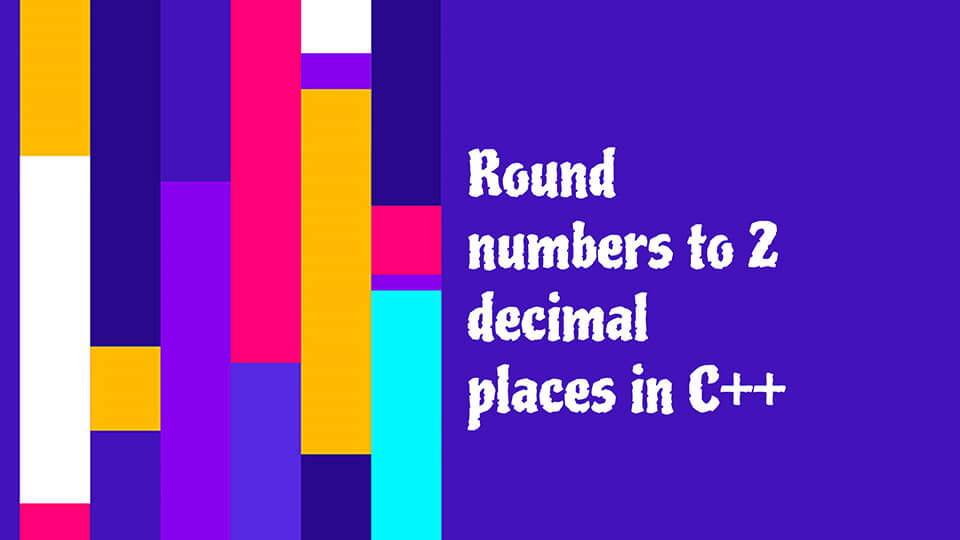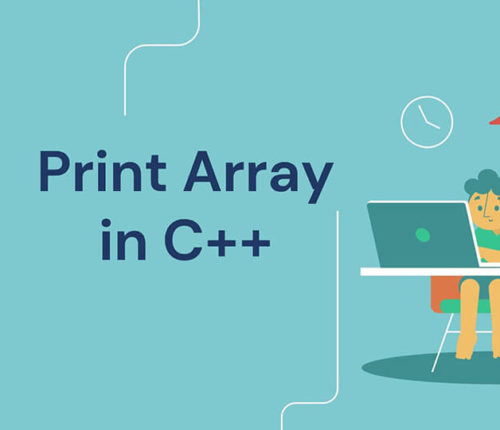# Round to 2 decimal places in C++## Representing Decimal Numbers in C++

In C++, we can use `float` and `double` datatypes to represent decimal numbers. Each type has a specific size and range. The `float` type can have six digits precision at maximum and require four bytes of memory. The `double` type can have fifteen digits precision and need eight bytes of memory.

## Ways to round to 2 decimal places in C++

In this tutorial, we will discuss how to round to 2 decimal places in C++. These methods work with both `float` and `double` type variables.

### Using the `ceil()` function to round to 2 decimal places in C++

The `ceil()` function returns the smallest integer greater than the given integer. It will round up to the nearest integer. We can use this function to round to 2 decimal places in C++.

We will multiply the given number by 100 and pass it to this function. The output of this will be divided by 100, and eventually, we will get the original number rounded up to 2 decimal places.

For example,

Output:

5.83

### Using the `floor()` function to round to 2 decimal places in C++

The `floor()` function returns the largest integer smaller than the given integer. It will round down to the nearest integer.

We will use this function as we did in the previous function. The original number will be rounded down to 2 decimal places.

See the code below.

Output:

5.82

### Using the `int` typecast to round to 2 decimal places in C++

We can typecast variables to an integer value using the `int` function. After typecasting, we can use the logic demonstrated in the previous two methods to carry out the rounding of numbers to 2 decimal places.

We will add `0.5` value to the converted value to round up the number.

For example,

Output:

5.82

### Using the `std::setprecision()` function to round to 2 decimal places in C++

This method is useful for displaying output with the desired number of precision places. The `setprecision()` function is a part of the `iomanip` header. It specifies the precision in the standard stream. The number of digits is provided as an argument for this function.

To view numbers with 2 decimal places in C++, we provide 3 as the argument of this function.

For example,

Output:

5.82

### Using the `printf()` function to round to 2 decimal places in C++

We use the `printf()` in C++ to display strings in the desired format. It is a part of the `cstdio` header file. We use the `%.2f ` format specifier to display values rounded to 2 decimal places.

See the code below.

Output:

5.82

### Using the `fprintf()` function to round to 2 decimal places in C++

The `fprintf()` function is similar to the `printf()` function but can also write to any File stream object. To display rounded numbers, we specify the `stdout` stream within this function.

For example,

Output:

5.82

## Conclusion

In this article, we discussed several methods to round to 2 decimal places in C++. We can use the `ceil()` and `floor()` function from the `cmath` header file. We can typecast decimal to integer and then convert it by performing some calculation. We can set the precision digits of the output stream with the `setprecision()` function. We can use the `printf()` and `fprintf()` function to round numbers to 2 decimal places by providing the `%.2f` format specifier.

## Related Posts

•19 May

### Get Type of Object in C++

Table of ContentsWhy Should We Determine the Type of Object in C++: Dynamic DispatchingGet Type of Object in C++Conclusion C++ is an object-oriented language where we often interact with objects of different types. The object interaction becomes more interesting when we add runtime polymorphism to it. The runtime polymorphism, also known as, dynamic dispatch of […]

•19 May

### Convert Vector to Array in C++

Table of ContentsVectors and Arrays in C++Convert Vector to Arrays in C++Using the for loop to convert vector to array in C++Using the copy() function to convert vector to array in C++Using the transform() function to convert vector to array in C++Using the data() function to convert vector to array in C++Using the & operator […]

•23 April

### How to initialize an Array in Constructor in C++

Table of ContentsArraysConstructors of a ClassWays to initialize an array in Constructor in C++Initialize an array in Constructor With std::fill()Initialize an array in Constructor Using for LoopInitialize an array in Constructor Using Member Initializer ListConclusion This article discusses methods of initializing an array in constructor in C++. Let us first discuss arrays and constructors in […]

•31 March

### Write String to File in C++

Table of ContentsUsing the fstream Library to write String to File in C++Using the Insertion Operator (<<) With the fstream ObjectUsing the write() Function of the fstream LibraryUsing the fwrite() Function to write String to File in C++Conclusion The files are used to store the data permanently on a disk. This mitigates the problem of […]

•23 March

### Print Array in C++

Table of ContentsIntroduction of ArraysDifferent Methods to Print an Array in C++print array in C++ by Traversing Indicesprint array in C++ Using for_each() Functionprint array in C++ Using Range-Based for Loopprint array in C++ Using Iteratorsprint array Using ostream_iterator() Function and copy() Functionprint array Using C++17 copy() Function and make_ostream_joiner() FunctionConclusion An array is a […]

•23 March

### Print Vector in C++

Table of ContentsPrint Vector in C++ Using IndicesPrint Vector in C++ Using Range-Based for LoopPrint Vector in C++ Using for_each() FunctionPrint Vector in C++ Using IteratorPrint Vector in C++ by Overloading the Insertion Operator (<<)Print Vector in C++ Using copy() FunctionConclusion This article discusses the vector and how to print vector in C++. Vectors are […]

## Subscribe to our newletter

Get quality tutorials to your inbox. Subscribe now.